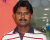# venkatc

Members

61

## Everything posted by venkatc

1.## Different Font Size For Data Labels

Please find the attachment. I have given the description in the screen shot. Please suggest.
2.## 0 Decimal Points In Y-Axis In Mscolumn2D.swf

I am working with some older version of Fusion Charts (not v3.2). I am using MSColumn2D.swf to render the graph. My requirement is to display one decimal values in Y-Axis. This is working fine for decimals other than zero. For example if the number is 10 or 10.0 I would like to display 10.0 only in Y-Axis. But with the older version it is displaying as 10 not as 10.0. Can you please advise how can I fix the issue. I even tried the below chart attributes but it is not working. <chart yAxisMinValue='115' yAxisMaxValue='140' adjustDiv='0' numDivLines='3' forceYAxisValueDecimals='1' yAxisValueDecimals='2' decimals='0' > Thanks
3.## 0 Decimal Points In Y-Axis In Mscolumn2D.swf

Thanks for the reply. But the code you suggested still does not show zero decimal. I am using the below xml for old version of fusion chart. <chart yAxisMinValue='115' yAxisMaxValue='140' adjustDiv='0' numDivLines='3' forceYAxisValueDecimals='1' yAxisValueDecimals='1' decimals='1' formatNumberScale='0'> <categories> <category label="1-Year"/> <category label="3-Year"/> <category label="5-Year"/> </categories> <dataset seriesName="AA" color="669900" > <set value="45.5" displayvalue='45.5%' color="#c0504d"/> <set value="13.3" displayvalue='13.3%' color="#c0504d"/> <set value="7.8" displayvalue='7.8%' color="#c0504d"/> </dataset> <dataset seriesName="Peer Median" color="3399FF" > <set value="47.2" displayvalue='47.2%'/> <set value="13.8" displayvalue='13.8%'/> <set value="8.0" displayvalue='8.0%'/> </dataset> <dataset seriesName="Industry Median" color="454545" > <set value="40.0" displayvalue='40.0%'/> <set value="-8.6" displayvalue='-8.6%'/> <set value="0.0" displayvalue='0.0%'/> </dataset> </chart>
4. Hi , We are using MSLine2D chart, Some times data points are overlapping with other data points. Can you please advice how to solve this problem. Please find the attached file for your reference. Thanks, Venkat
5. Is it possible to put an image as label in Trend Line.
6. Is it possible to fix the Canvas Height.
7. Is it possible to customize the Font Color and Font Size in TrendLine Label.
8. Hi All, We are using MSColumn3D chart to display the data. For first columns we are displaying 2 different colors(Green,red).How can we show that two colors in legend. Please find the attached document for your reference and advice on this. Is it possible to create stacked legend ? Thanks, Venkat.
9. Hi All, We are trying to generate a chart which is shown in the attachment. Can please suggest the chart name. Thanks, Venkat
10. [p]Hi , We are using below xml to create water fall chart, but on the bar we want to display the text (Shown in image with red color circle). We used displayValue tag but the text is messedup with other bars text.(If the text is large it is touching other bars). Can anybody help us to formatting the display text on the bar(Shown in image with red color circle). Use attached image. chart caption='Year Over Year Change' yAxisName='Millions'numberPrefix='\$' exportHandler='fcExporter1' exportEnabled='1' exportAtClient='1' plotGradientColor='' DIVLINES='Animation' cumulative='0' showSumAtEnd='0' numdivlines='11' yAxisMinValue='0' yaxismaxvalue='22.0' outCnvBaseFontColor='#000000' bgColor='#ffffff' showAlternateHGridColor='0' showFCMenuItem='0' set label='2009' color='#F79646' font="16" Value='14.1' set value='0.3' color='#376092' set value='0.3' width='10' color='#376092' set value='3' color='#376092' set value='2' color='#376092' set label='2010' isSum='1' color='#F79646' font="16" cumulative='1' chart Thanks, Venkat.[/p]